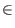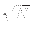Next: How Many Iterations are Up: Quantum Computing and Grover's Previous: A Special Case   Contents

# Open Questions

There are several open questions in Grover's paper. Foremost among these is how many times exactly should we iterate step 2 of Grover's algorithm. Grover proves the existence of some mO(), such that after m iterations of step 2 of the algorithm the probability of finding the register in the marked state is greater than 1/2. Since the amplitude of the desired state, and hence the of probability of measuring the desired state, is not monotonic increasing after m iterations, it is not enough to know know the existence of m, it's value must be determined.

SubsectionsNext: How Many Iterations are Up: Quantum Computing and Grover's Previous: A Special Case   Contents
Matthew Hayward - Quantum Computing and Grover's Algorithm GitHub Repository